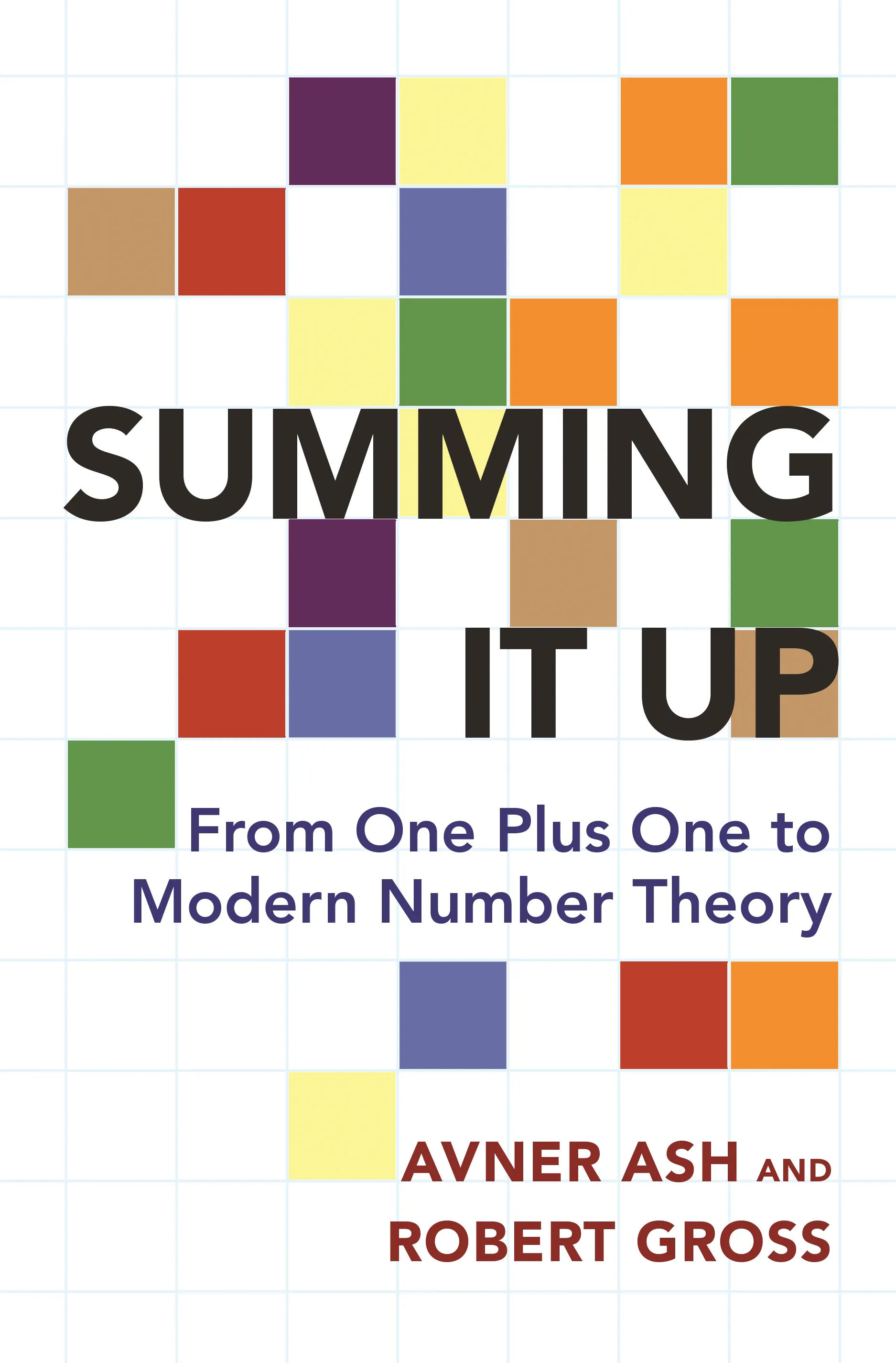#### Summing It Up

Avner Ash

##### ebooks

Many of our ebooks are available for purchase from these online vendors:

Many of our ebooks are available through library electronic resources including these platforms:

# Summing It Up: From One Plus One to Modern Number Theory

The power and properties of numbers, from basic addition and sums of squares to cutting-edge theory## Paperback

Price:
\$16.95 / £12.99
ISBN:
Published:
Jan 30, 2018
2018
Pages:
248
Size:
6 x 9.25 in.
Illus:
16 b/w illus., 4 tables

We use addition on a daily basis—yet how many of us stop to truly consider the enormous and remarkable ramifications of this mathematical activity? Summing It Up uses addition as a springboard to present a fascinating and accessible look at numbers and number theory, and how we apply beautiful numerical properties to answer math problems. Mathematicians Avner Ash and Robert Gross explore addition’s most basic characteristics as well as the addition of squares and other powers before moving onward to infinite series, modular forms, and issues at the forefront of current mathematical research.

Ash and Gross tailor their succinct and engaging investigations for math enthusiasts of all backgrounds. Employing college algebra, the first part of the book examines such questions as, can all positive numbers be written as a sum of four perfect squares? The second section of the book incorporates calculus and examines infinite series—long sums that can only be defined by the concept of limit, as in the example of 1+1/2+1/4+…=? With the help of some group theory and geometry, the third section ties together the first two parts of the book through a discussion of modular forms—the analytic functions on the upper half-plane of the complex numbers that have growth and transformation properties. Ash and Gross show how modular forms are indispensable in modern number theory, for example in the proof of Fermat’s Last Theorem.

Appropriate for numbers novices as well as college math majors, Summing It Up delves into mathematics that will enlighten anyone fascinated by numbers.